#### Number of problems found: 1307

• Common divisorsFind all common divisors of numbers 30 and 45.
• Colza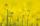In the agricultural cooperative harvested 525 ares of colza, of which received 5.6 tons of seeds. Calculate the yield per hectare.
• Christmas napkinsThe girls embroidered Christmas napkins. Each napkin had the shape of a triangle with sides of 5 dm, 60 cm, and 800 mm. How many cms did the girls sew if they made 15 napkins?
• Triangular prismCalculate a triangular prism if it has a rectangular triangle base with a = 4cm and hypotenuse c = 50mm and height of the prism is 0.12 dm.
• Saving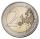The boy has saved 50 coins € 5 and € 2. He saved € 190. How many were € 5 and how many € 2?Add marks (+, -, *, /, brackets) to fullfill equations 1 3 6 5 = 10 This is for the 4th grade of the primary school - with no negative numbers yet
• Rings - intersect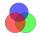There are 15 pupils on the sporting ring. 10 pupils go to football, 8 pupils go to floorball. How many pupils go to both rings at the same time?
• EquationFind x: x + 1/2 = 1/3
• Moneys in triple ratioMilan, John and Lili have a total 344 euros. Their amounts are in the ratio 1:2:5. Determine how much each of them has?
• Bicycle gears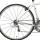The toothed wheel on the bicycle pedal has 40 teeth, the wheel on the rear wheel has only 16 teeth. How many times does the rear wheel turn if the pedals rotate 50 times?
• Perimeter to areaCalculate the area of a circle with the perimeter 15 meters.
• Cube edgeDetermine the edges of the cube when the surface is equal to 37.5 cm square.
• PointsGryffindor won 437 points. How many points obtained by each of the faculties if they were split at a ratio of 5: 7: 3: 4?
• Lcd3What is LCD of the equation of ? ? And what is x?
• Expression plus minusEvaluate expression: (-1)2 . 12 – 6 : 3 + (-3) . (-2) + 22 – (-3) . 2
• Iron pole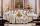What is the mass of pole with the shape of a regular quadrilateral prism with a length of 1 m and a cross-sectional side length of a = 4.5 cm make from iron with density ρ = 7800 kg/m³?
• LCM of two numberFind the smallest multiple of 63 and 147
• BuildingAt the building, we divided 240 boards into two piles in a 5: 3 ratio. How many were fewer boards in the lower pile?
• Farmers 2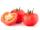On Wednesday the farmers at the Grant Farm picked 2 barrels of tomatoes. Thursday, the farmers picked 1/2 as many tomatoes as on Wednesday. How many barrels of tomatoes did the farmers pick on Thursday?
• DivideDivide substance 110 cm long to two parts so that it first part is 10 cm longer than the second part and one part will be 10 times longer than the second portion. How long the parts will be?

Do you have an interesting mathematical word problem that you can't solve it? Submit a math problem, and we can try to solve it.

We will send a solution to your e-mail address. Solved examples are also published here. Please enter the e-mail correctly and check whether you don't have a full mailbox.

Please do not submit problems from current active competitions such as Mathematical Olympiad, correspondence seminars etc...